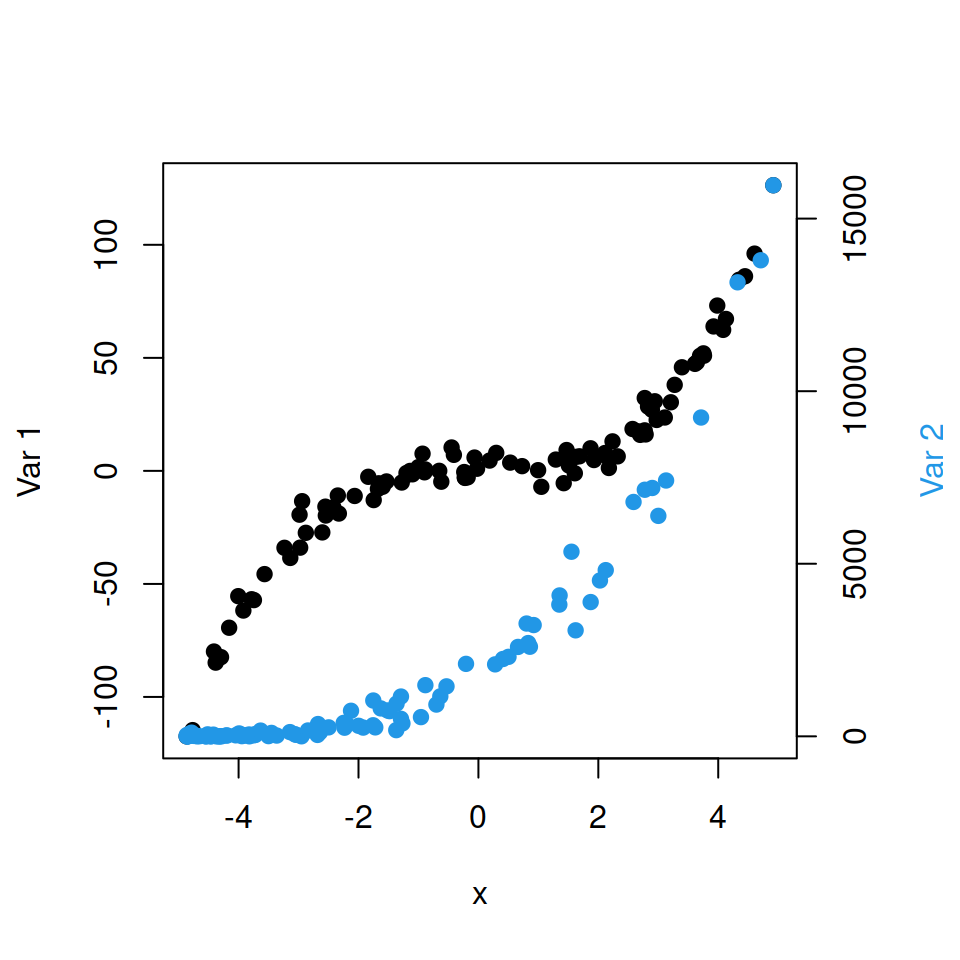# Axes customization in R

We are going to use the data of the code above on the following examples:

``````# Reproducible data
set.seed(1)
x <- runif(100, min = -5, max = 5)
y <- x ^ 3 + rnorm(100, mean = 0, sd = 5)``````

## X and Y axis labels

The default axis labels will depend on the function you are using, e.g. `plot` function will use the names of the input data, `boxplot` won’t show any axis labels by default and `hist` will show the name of the variable on the X-axis and “Frequency” or “Density” on the Y-axis, depending on the type of the histogram.

Default plot

For illustration purposes we are going to use the `plot` function but the examples are extensible to other functions. In order to plot the data we defined before you can type:

``plot(x, y, pch = 19)``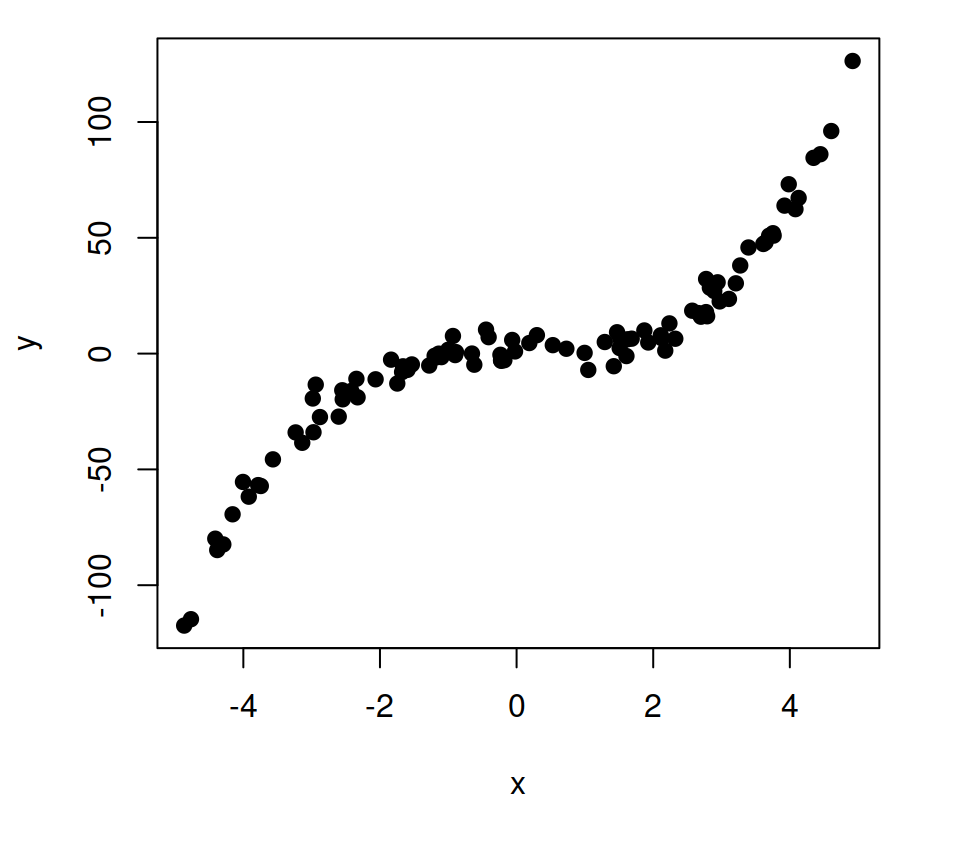Custom axes labels

In order to change the axis labels you can specify the arguments `xlab` and `ylab` as follows:

``````plot(x, y, pch = 19,
xlab = "My X-axis label",
ylab = "My Y-axis label")``````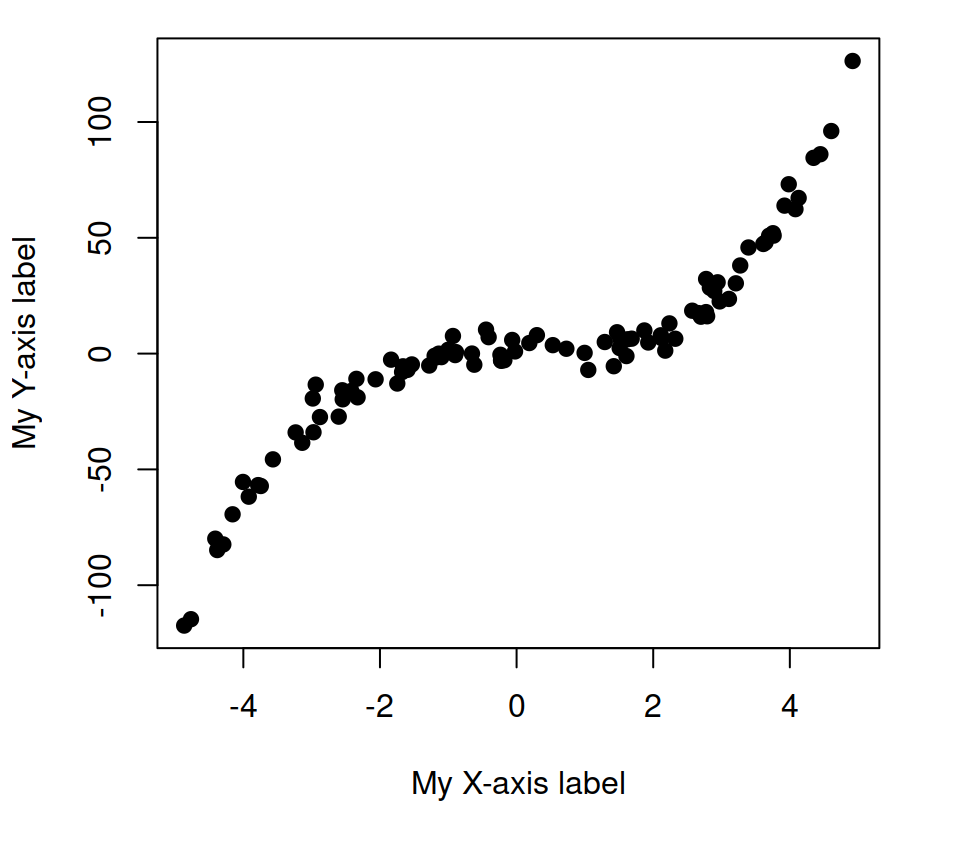### Labels color

In addition, is is possible to modify the color of the axes labels with the `col.lab` argument.

``````plot(x, y, pch = 19,
xlab = "My X-axis label",
ylab = "My Y-axis label",
col.lab = 4) # Color of the labels``````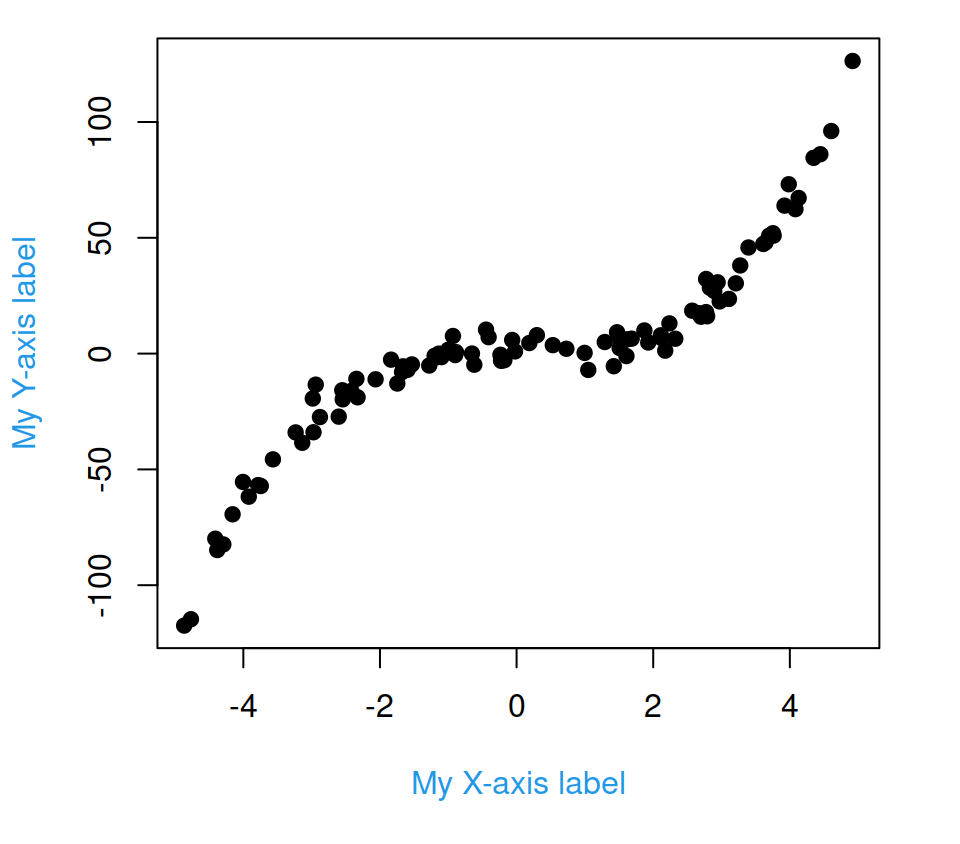### Remove axis labels

You can remove the axis labels with two different methods: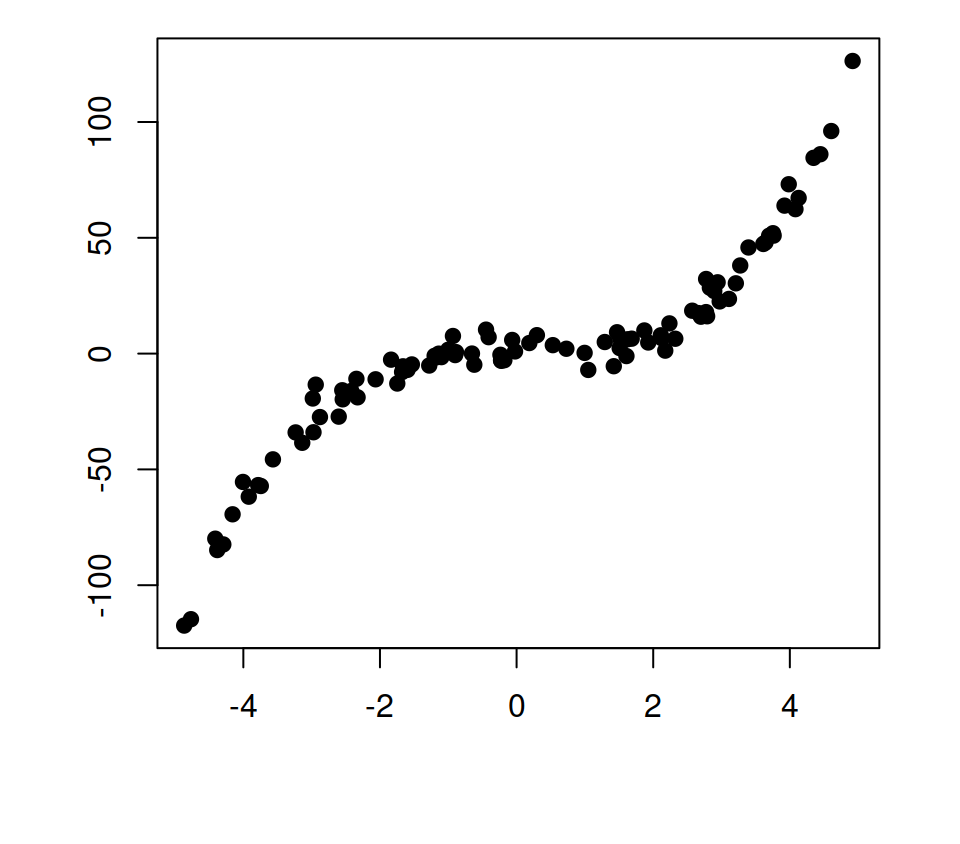Option 1. Set the `xlab` and `ylab` arguments to `""`, `NA` or `NULL`.

``````# Delete labels
plot(x, y, pch = 19,
xlab = "", # Also NA or NULL
ylab = "") # Also NA or NULL``````Option 2. Set the argument `ann` to `FALSE`. This will override the label names if provided.

``````# Equivalent
plot(x, y, pch = 19,
xlab = "My X-axis label",
ylab = "My Y-axis label",
ann = FALSE)``````

## The `axis` function

The `axis` function allows adding axes to all sides of the the current plot, with the possibility of specifying the position and the labels of the tick marks. Recall to type `?axis` for further details.

axis Position
1 below
2 left
3 above
4 right

Option 1. Set `xaxt = "n"` and `yaxt = "n"` to remove the tick labels of the plot and add the new labels with the `axis` function. Note that the `at` argument sets where to show the tick marks.

``````plot(x, y, pch = 19,
xaxt = "n", yaxt = "n")

# X-axis
axis(1, at = c(-4, 0, 4))

# Y-axis
axis(2, at = c(-100, -50, 0, 50, 100))``````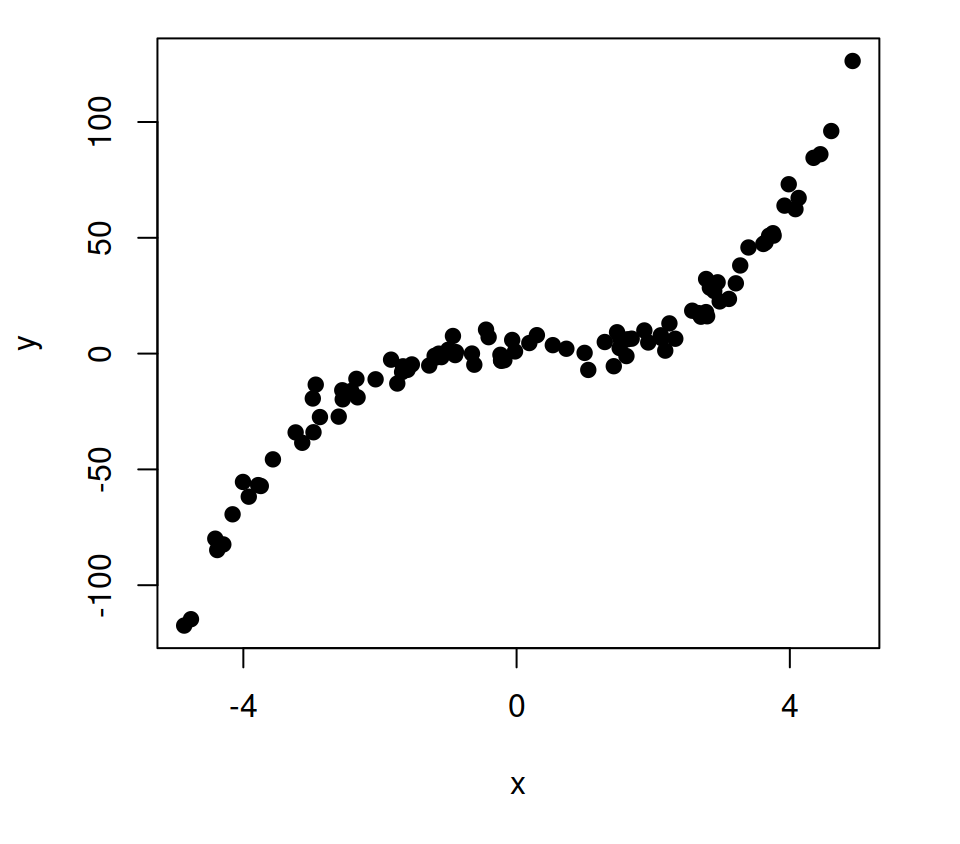Option 2. Set `axes = FALSE` inside your plotting function to remove the plot box and add the new axes with the `axis` function.

``````plot(x, y, pch = 19,
axes = FALSE)

axis(1)

axis(2)

# You can add the plot box again
# box()``````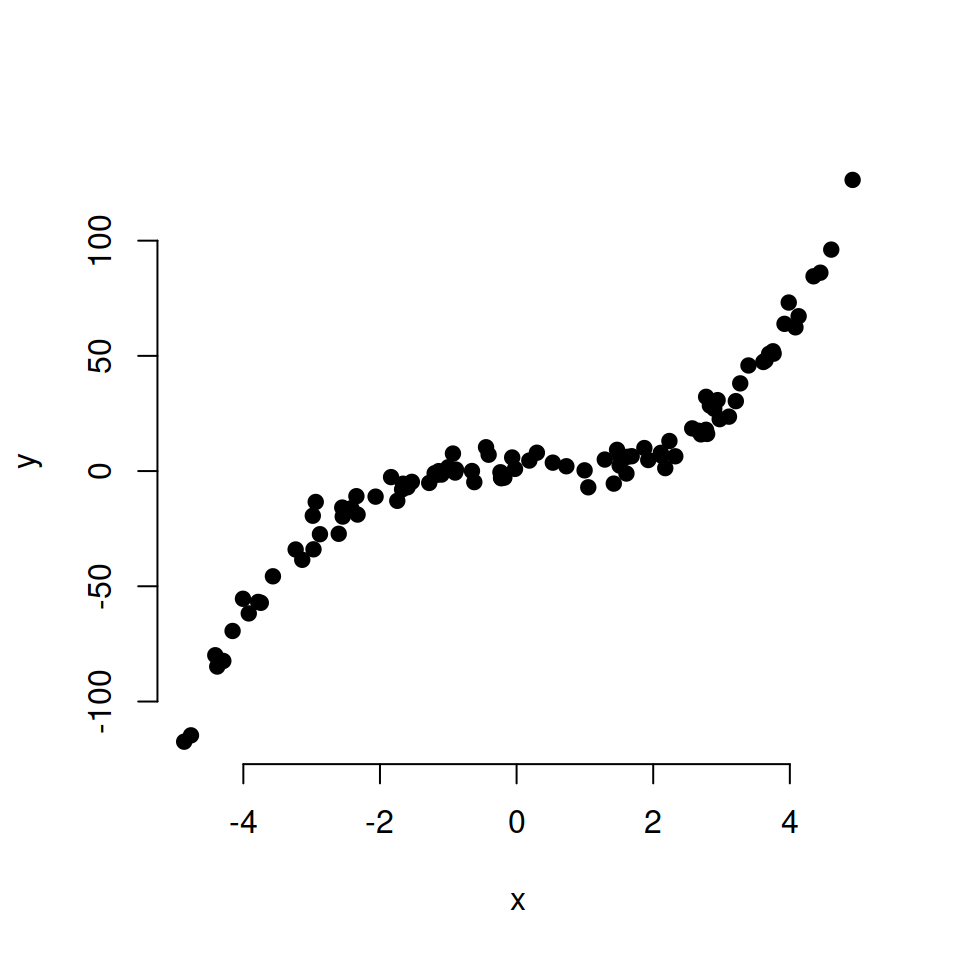Color of the axes

You can also customize the color of the axis and the ticks with the `col` and `col.ticks` arguments.

``````plot(x, y, pch = 19,
axes = FALSE)

axis(1,
col = "blue",        # Axis line color
col.ticks = "green", # Ticks color
col.axis = "red")    # Labels color

axis(2,
col = "blue",
col.ticks = "green",
col.axis = "red")``````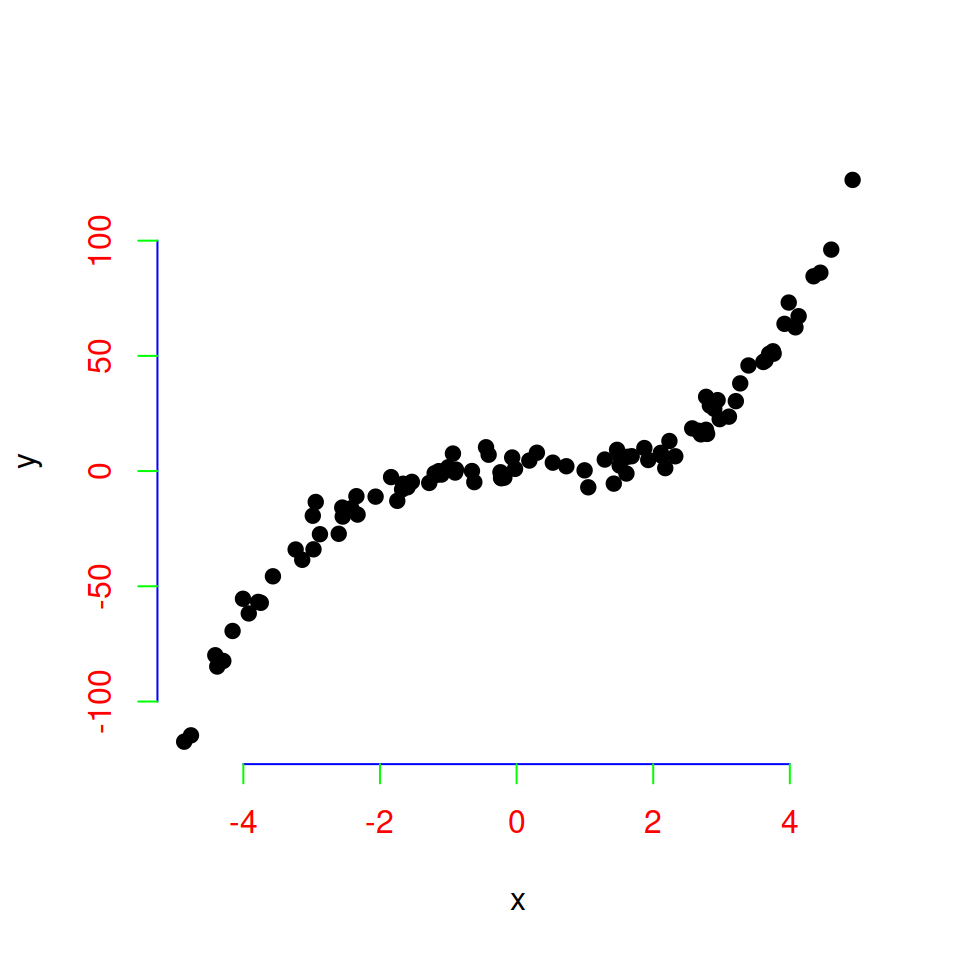## Axis tick marks

In addition to axes labels, the tick marks of each axis can be customized in different ways.

### `xaxp` and `yaxp` arguments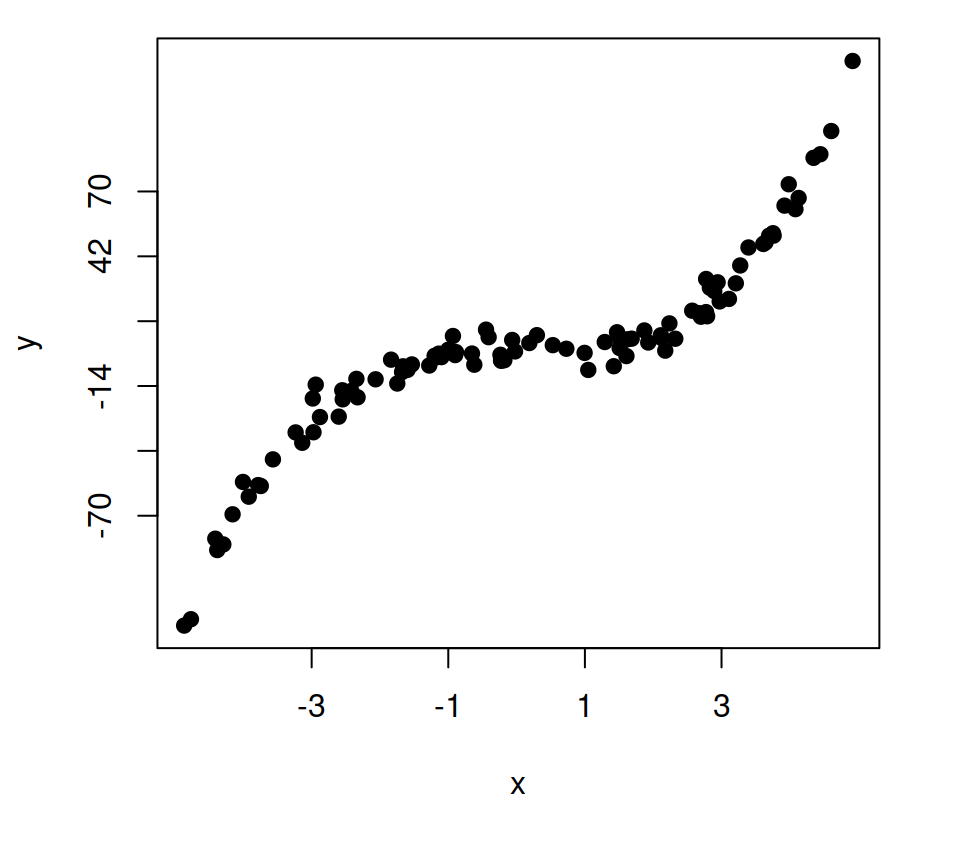The `xaxp` (X-axis) and `yaxp` (Y-axis) arguments allow customizing where the ticks of each axis start and end and the number of regions to divide the axis specifying vectors of the form `c(start, end, number_regions)`.

``````plot(x, y, pch = 19,
xaxp = c(-3, 3, 3),
yaxp = c(-70, 70, 5))``````

### Minor ticks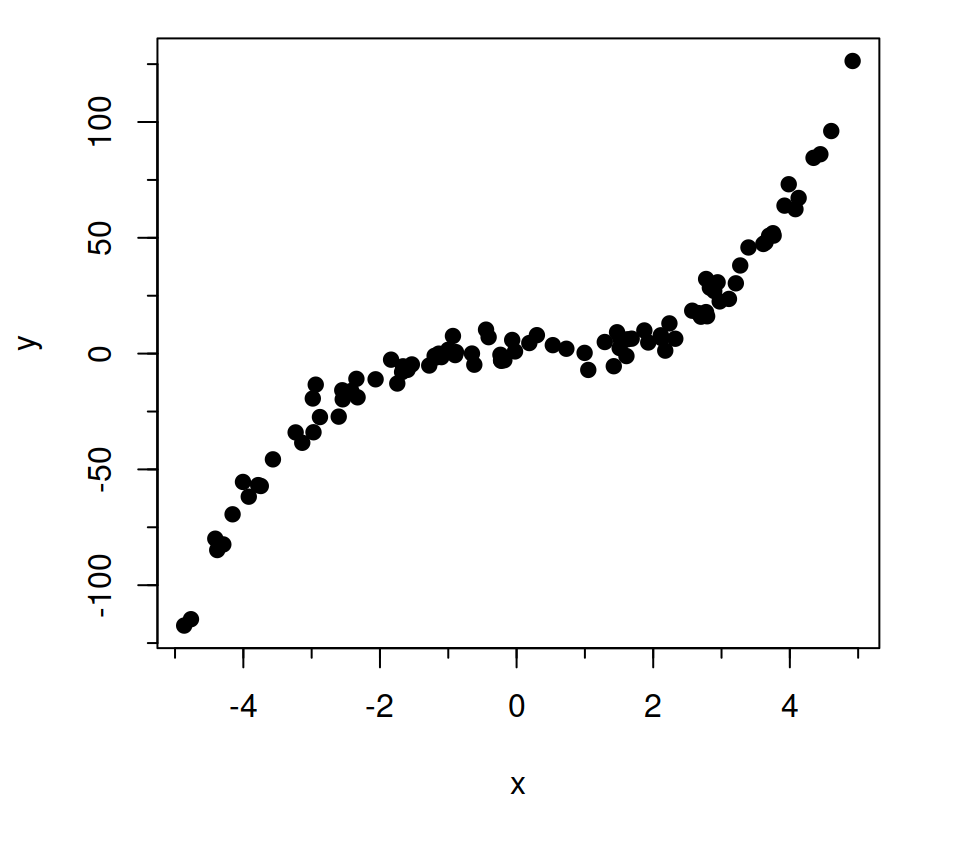It is possible to add minor ticks to the axes with the `minor.tick` function of the `Hmisc` library. The function will allow you to specify the tick density, the size and addition arguments to each axis.

``````# install.packages("Hmisc")
library(Hmisc)

plot(x, y, pch = 19)
minor.tick(nx = 2, ny = 2,   # Ticks density
tick.ratio = 0.5) # Ticks size``````

### Interior ticks

You can set interior ticks passing positive values to `tck` argument. The greater the value, the longer the ticks. Default value is `tck = -0.5`.

``````# Interior ticks
plot(x, y, pch = 19,
tck = 0.02)``````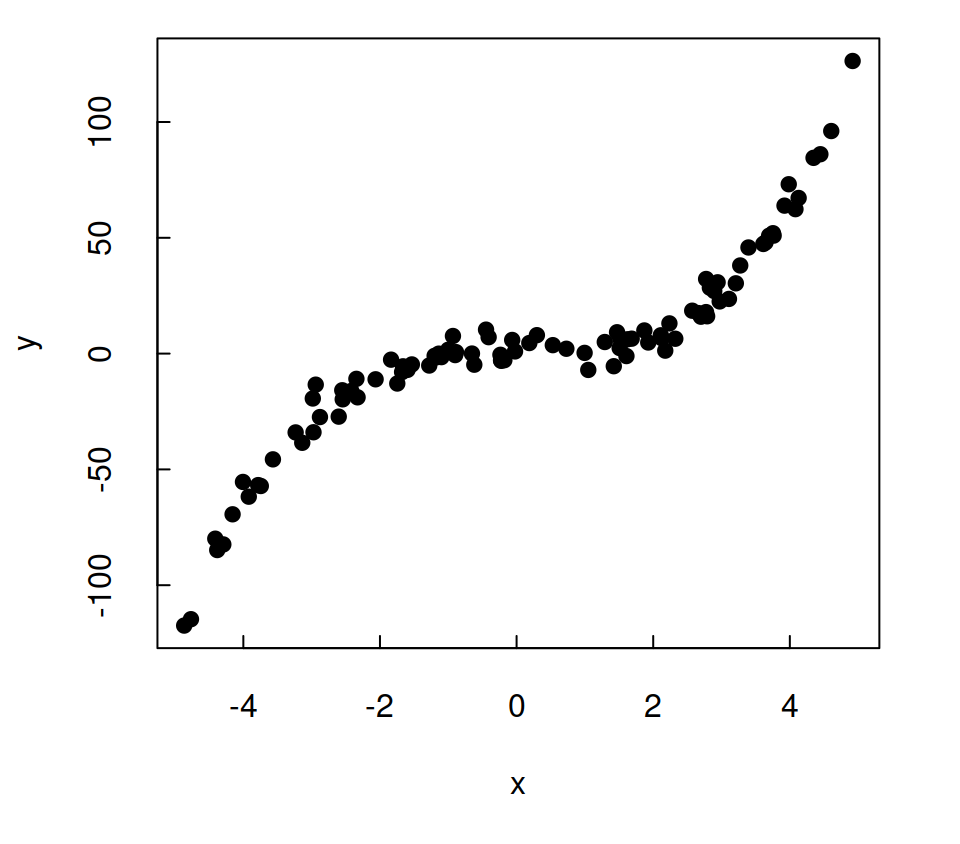### Rotate tick mark labels

It is possible to rotate the tick mark labels in several ways making use of the `las` argument.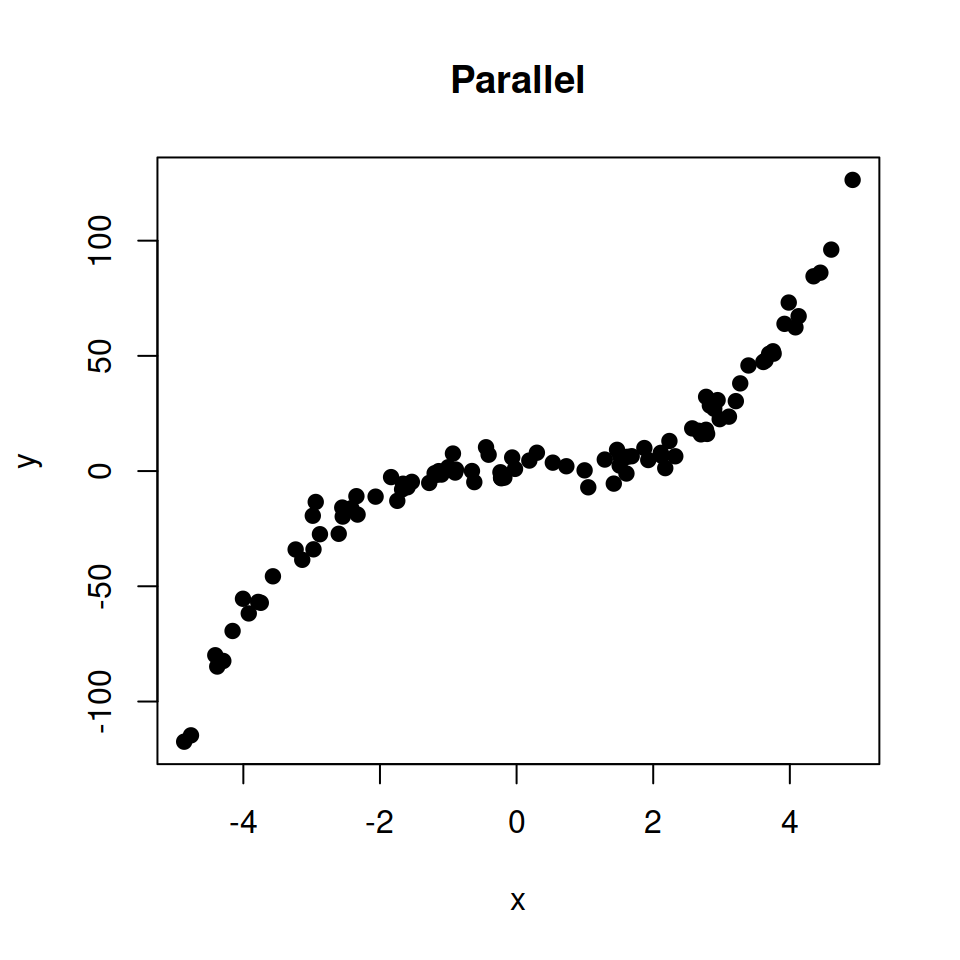Option 1. Parallel to axis (default).

``````plot(x, y, pch = 19,
las = 0,
main = "Parallel")``````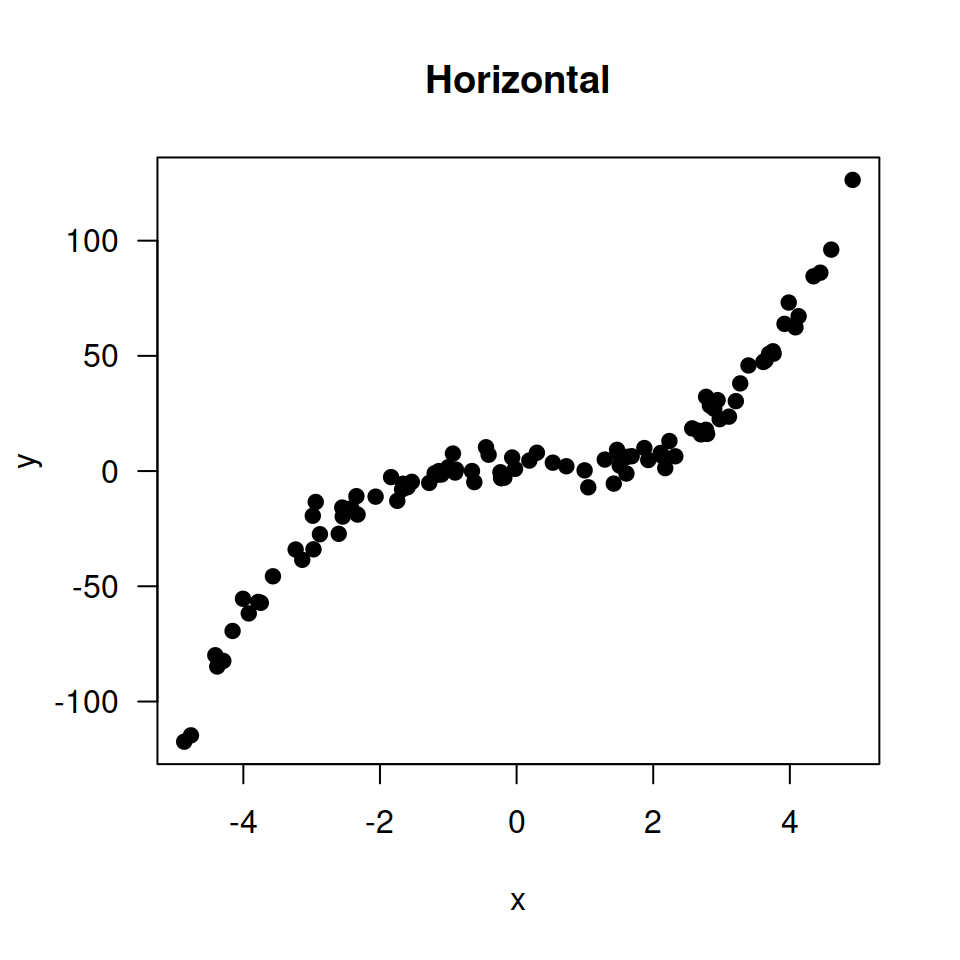Option 2. Horizontal.

``````plot(x, y, pch = 19,
las = 1,
main = "Horizontal")``````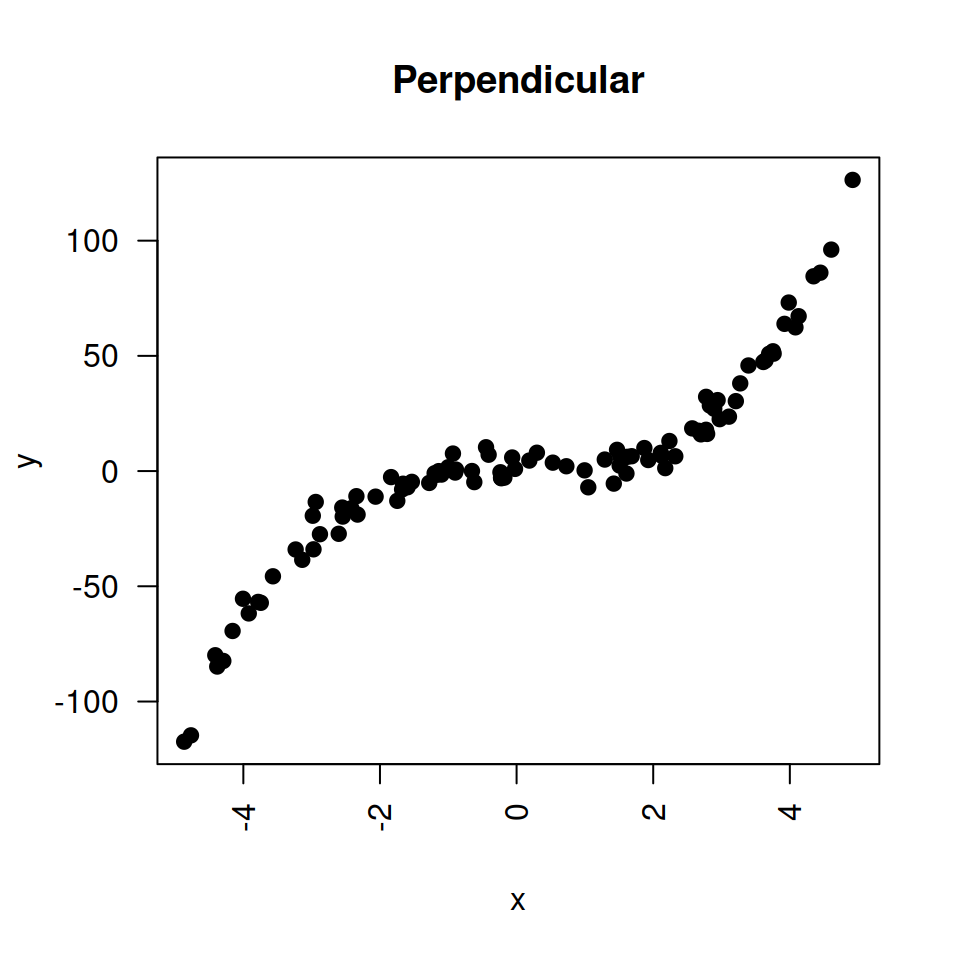Option 3. Perpendicular to axis.

``````plot(x, y, pch = 19,
las = 2,
main = "Perpendicular")``````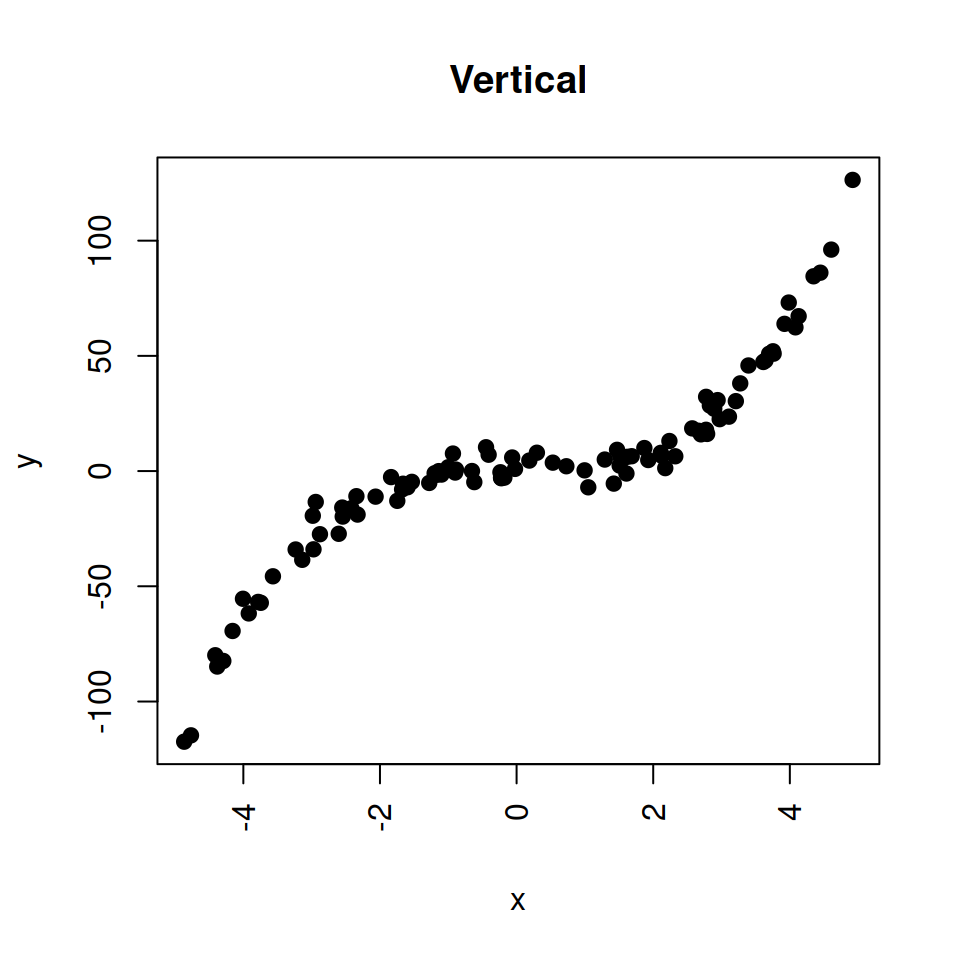Option 4. Vertical.

``````plot(x, y, pch = 19,
las = 3,
main = "Vertical")``````

### Custom tick mark labels

The `labels` argument of the `axis` function allows customizing the tick mark labels.

``````# Change X-axis tick labels
plot(x, y, pch = 19, xaxt = "n")
axis(1, at = seq(round(min(x)),
round(max(x)), by = 1),
labels = 1:11)``````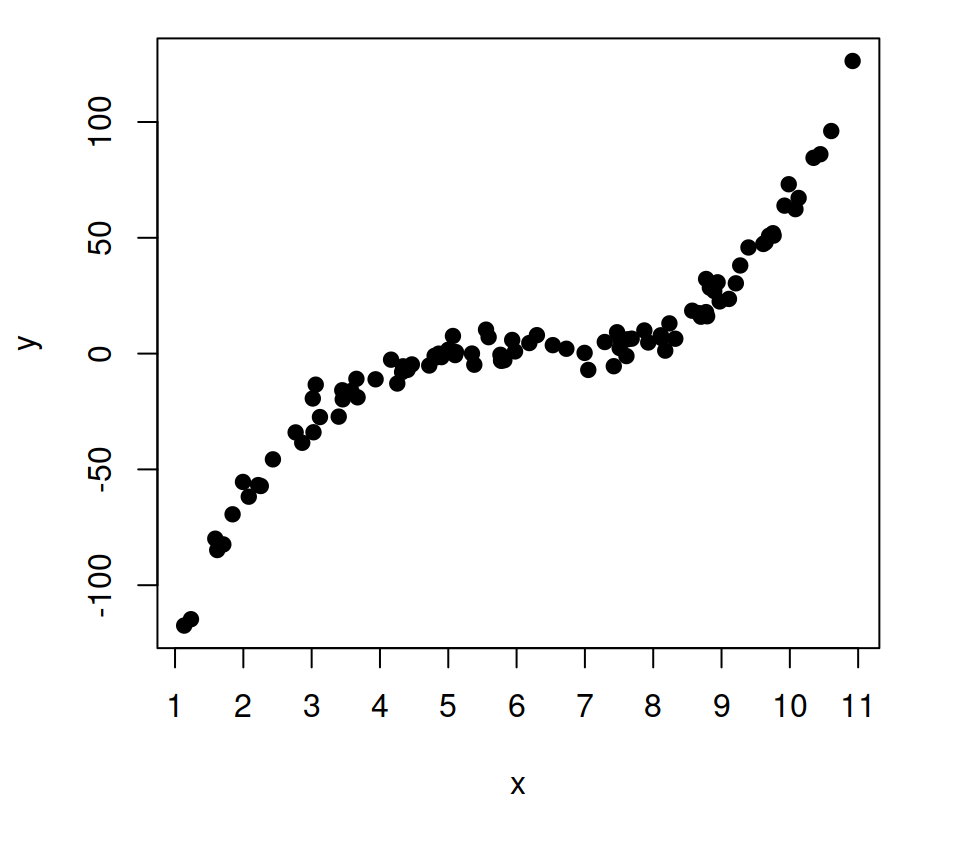Note that you can also set texts in addition to numbers, or even expressions.

``````# X-axis tick labels with text
plot(x, y, pch = 19, xaxt = "n")
axis(1, at = c(-2, 2),
labels = c("Some text", "Other text"))``````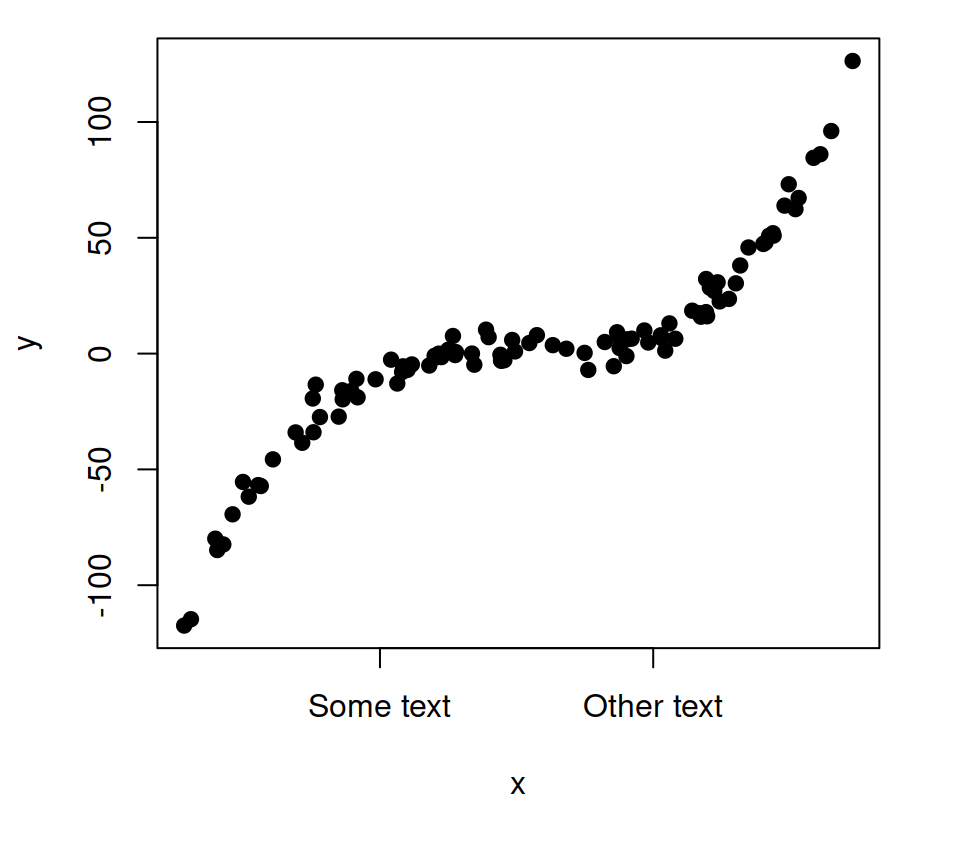### Remove tick marks

You can remove the tick marks of one of the two axis or of both at the same time setting arguments `xaxt` and `yaxt` to `""`.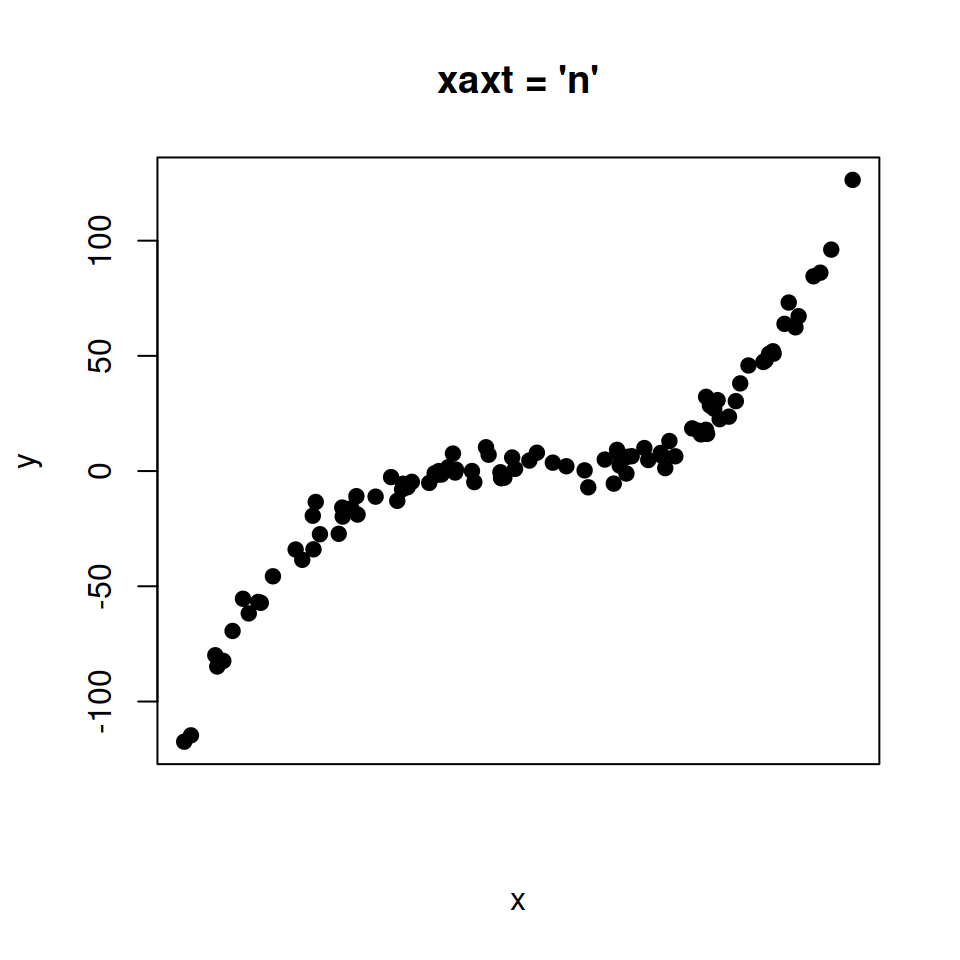Option 1. Remove only X-axis ticks.

``````# Remove X axis tick labels
plot(x, y, pch = 19,
xaxt = "n",
main = "xaxt = 'n'")``````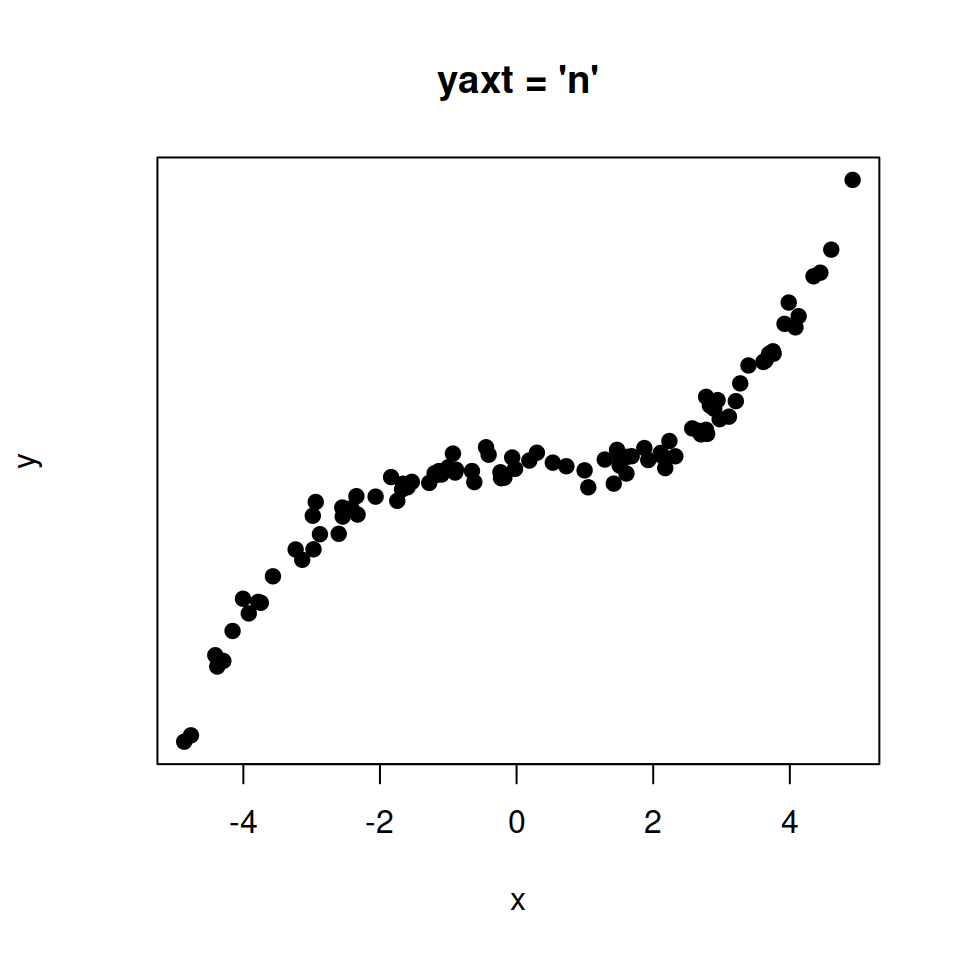Option 2. Remove only Y-axis ticks.

``````# Remove Y axis tick labels
plot(x, y, pch = 19,
yaxt = "n",
main = "yaxt = 'n'")``````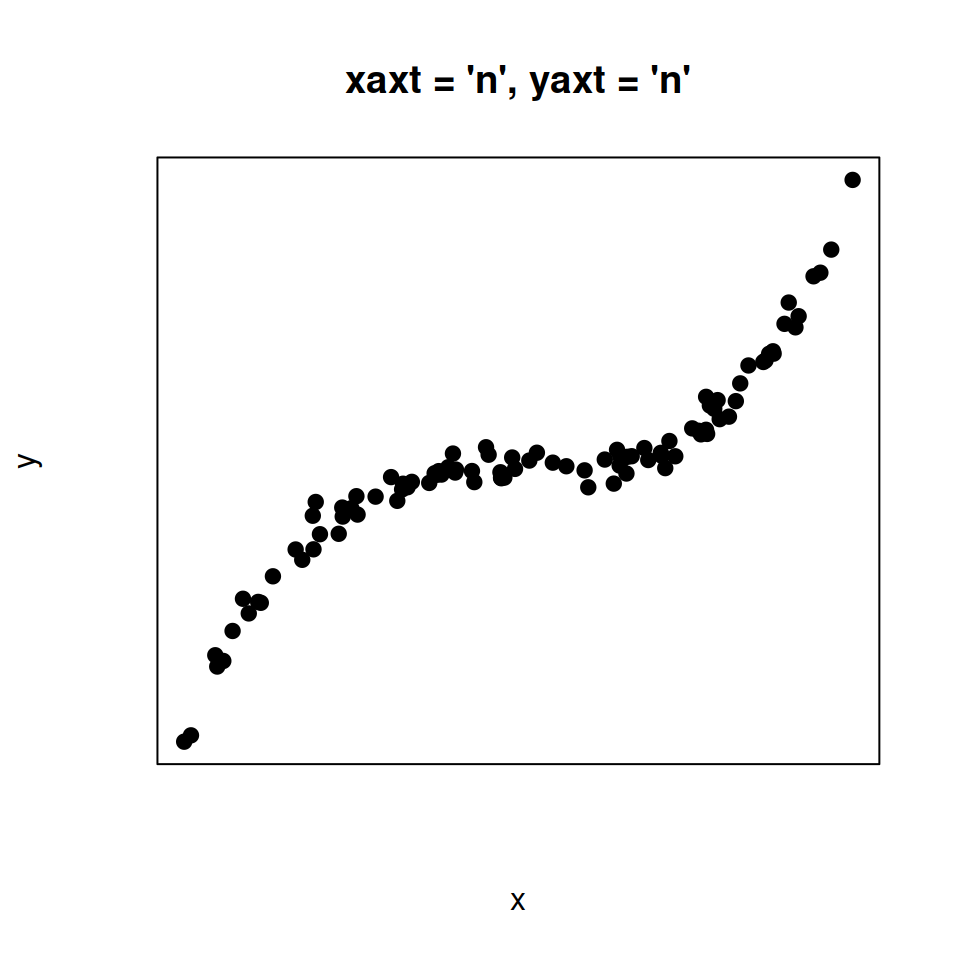Option 3. Remove both X-axis and Y-axis ticks.

``````# Remove both axis tick labels
plot(x, y, pch = 19,
yaxt = "n",
xaxt = "n",
main = "xaxt = 'n', yaxt = 'n'")``````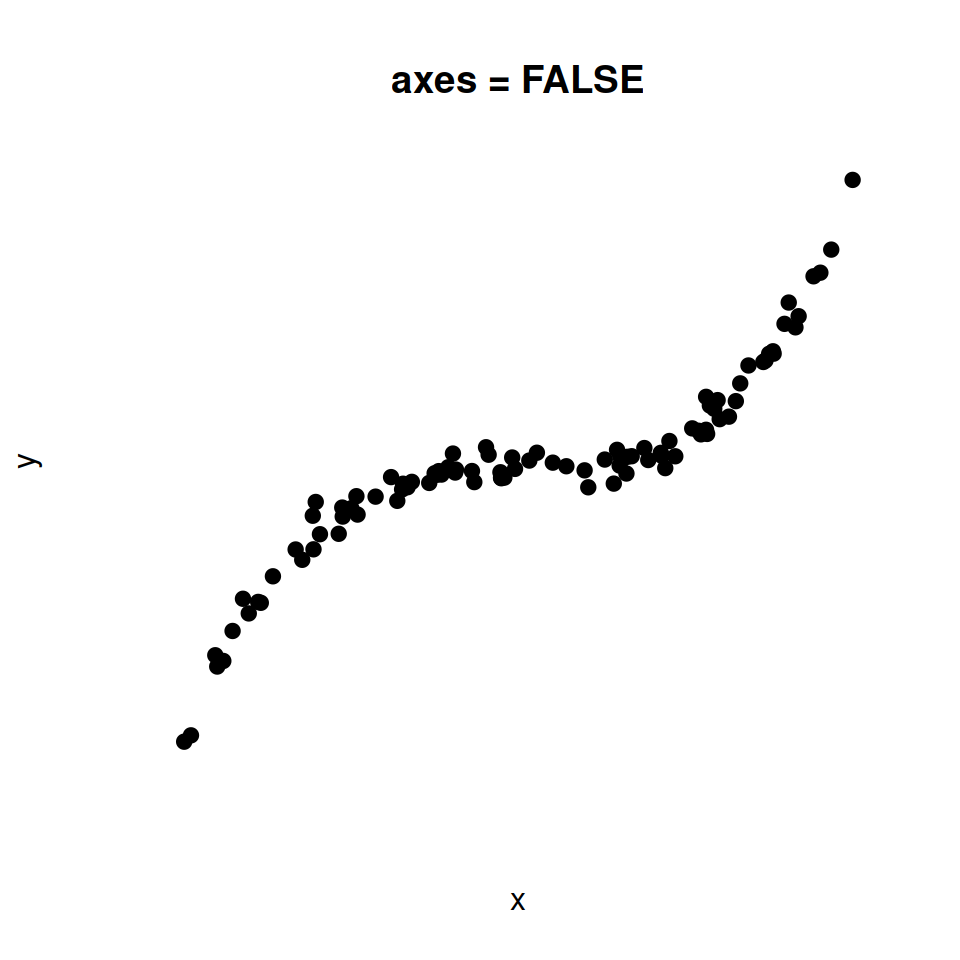Option 4. Remove both X-axis and Y-axis ticks and the box.

``````# Remove axis tick labels and the box
plot(x, y, pch = 19,
axes = FALSE,
main = "axes = FALSE")``````

## Axis limits

The axis limits can be customized with the `xlim` and `ylim` arguments for the X and Y axis, respectively.

``````# Axis limits
plot(x, y, pch = 19,
xlim = c(0, 4),   # X-axis limits
ylim = c(0, 100)) # Y-axis limits``````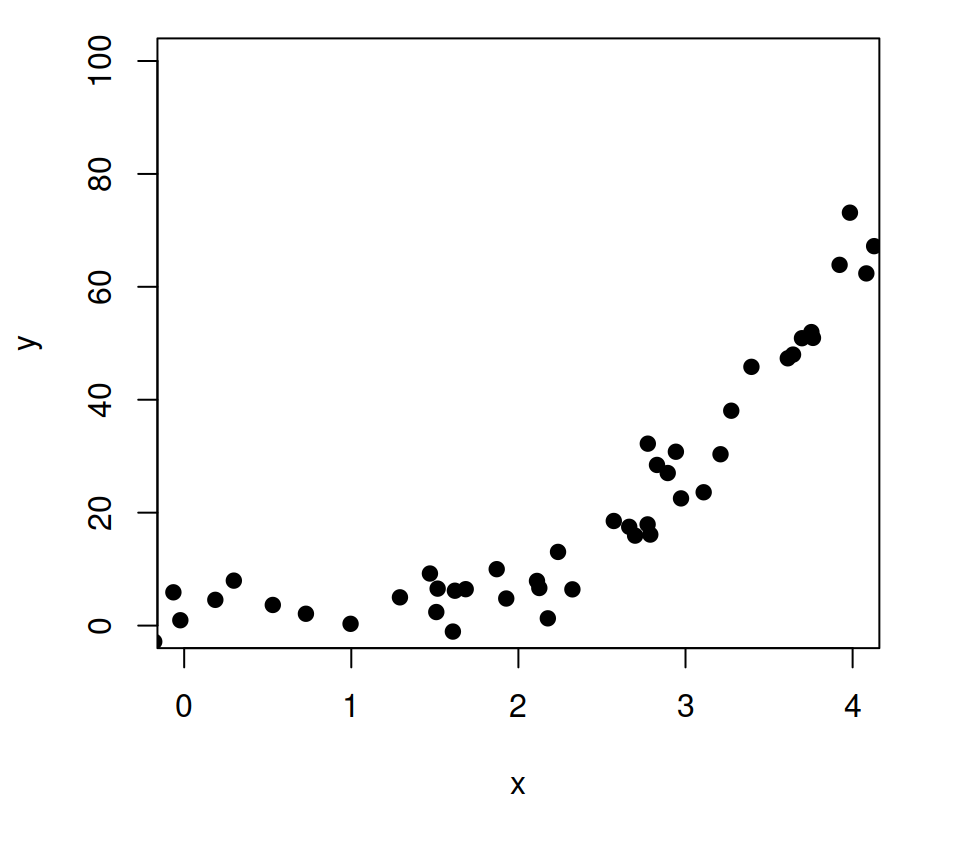## Axis scale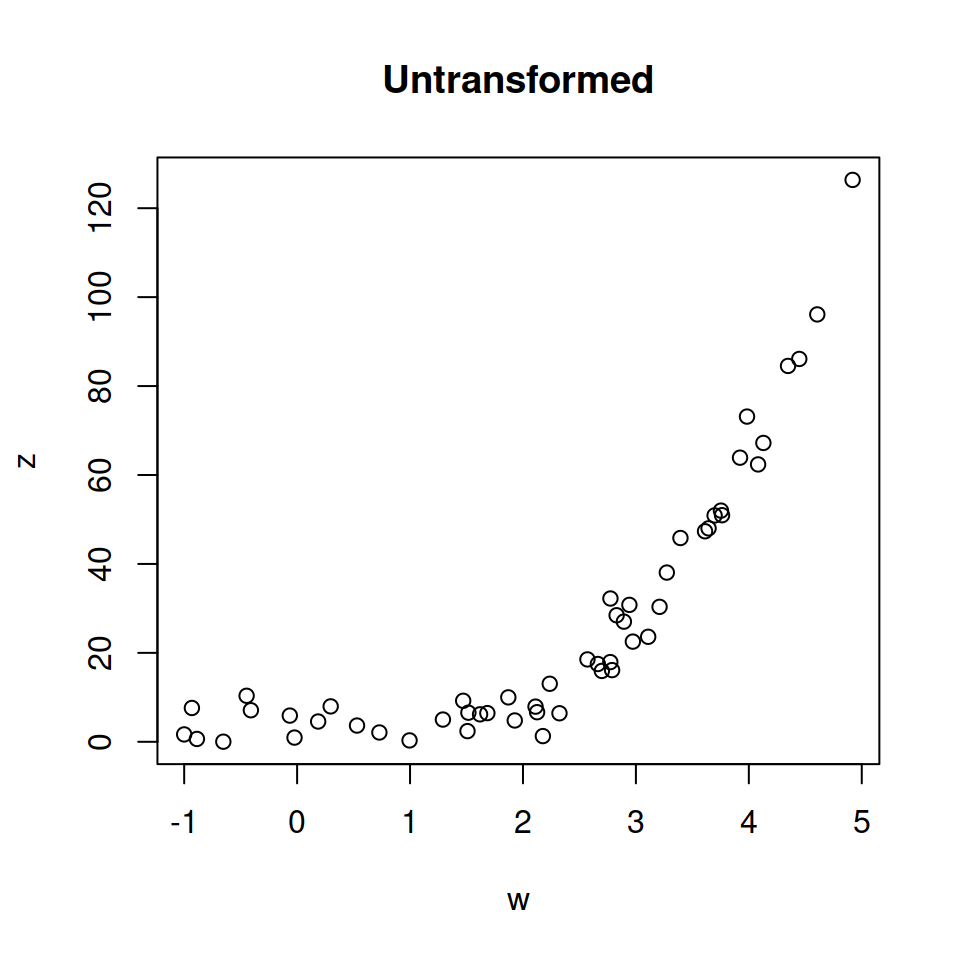Original data.

``````# Positive data
w <- x[x > 0]
z <- y[y > 0]

# Default
plot(w, z,
main = "Untransformed")``````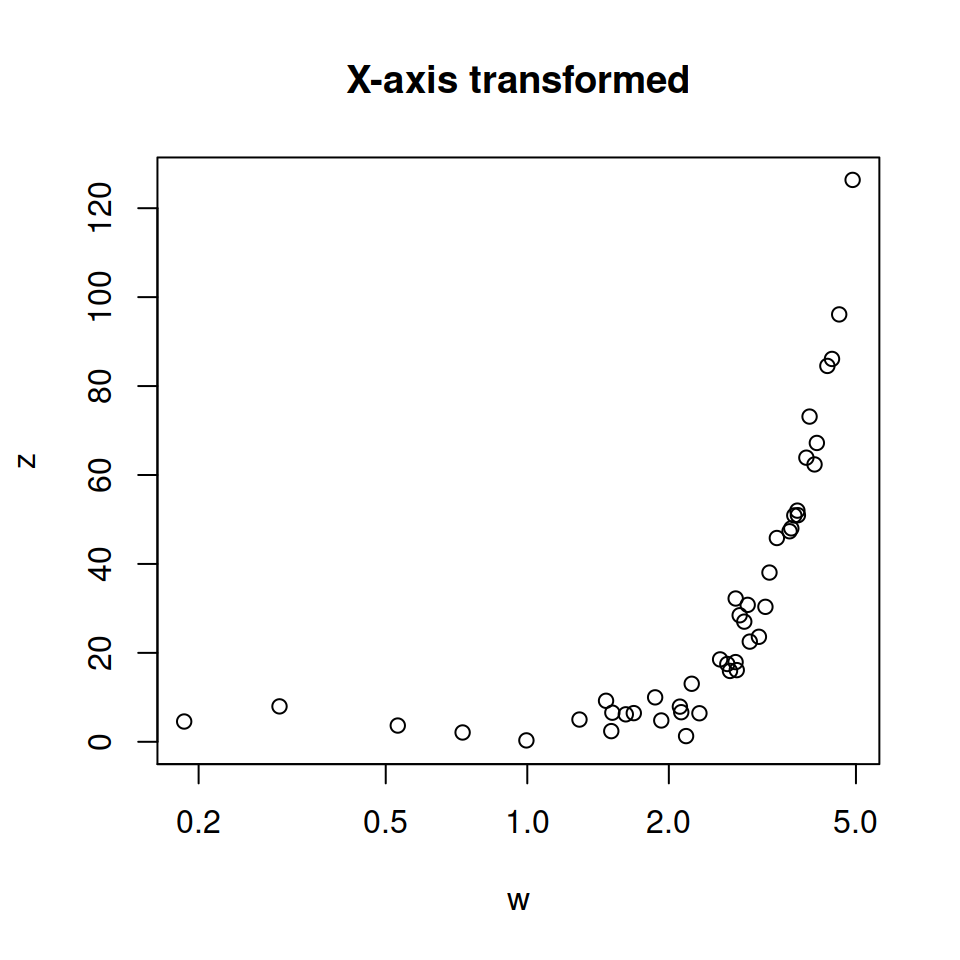X-axis transformed.

``````# Log scale. X-axis
plot(w, z,
log = "x",
main = "X-axis transformed")``````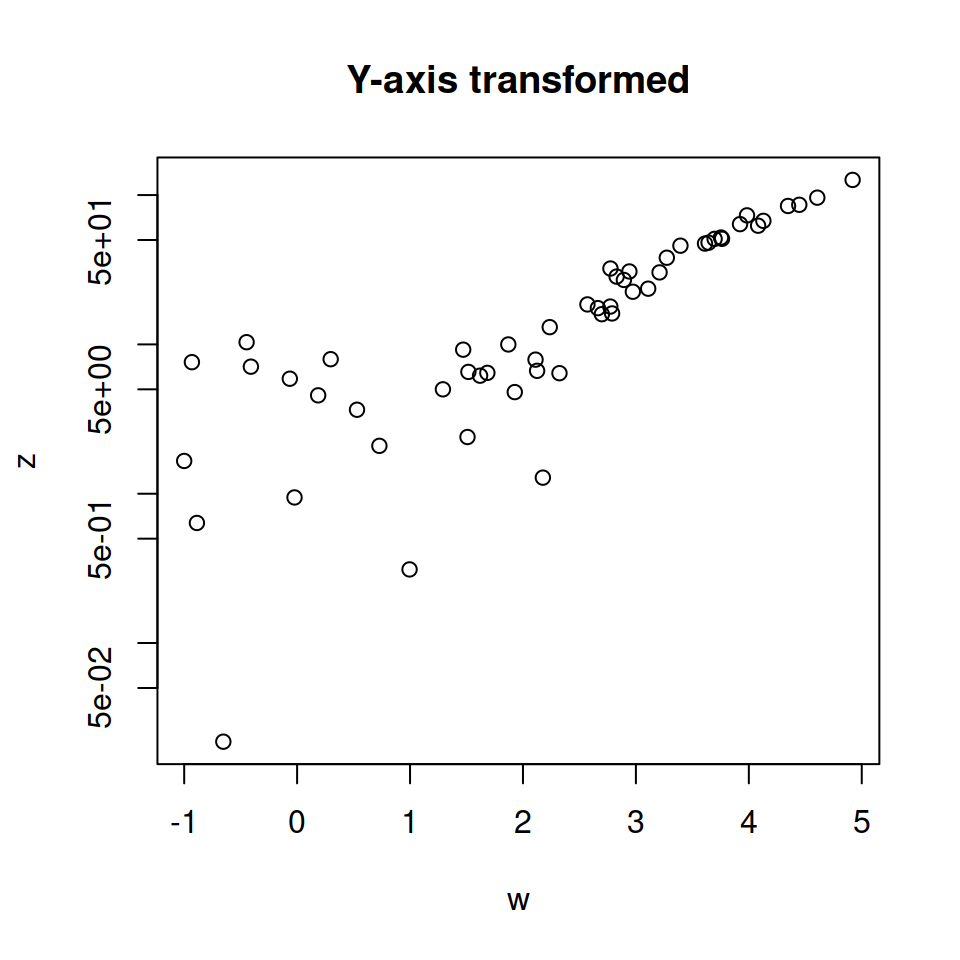Y-axis transformed.

``````# Log scale. Y-axis
plot(w, z,
log = "y",
main = "Y-axis transformed")``````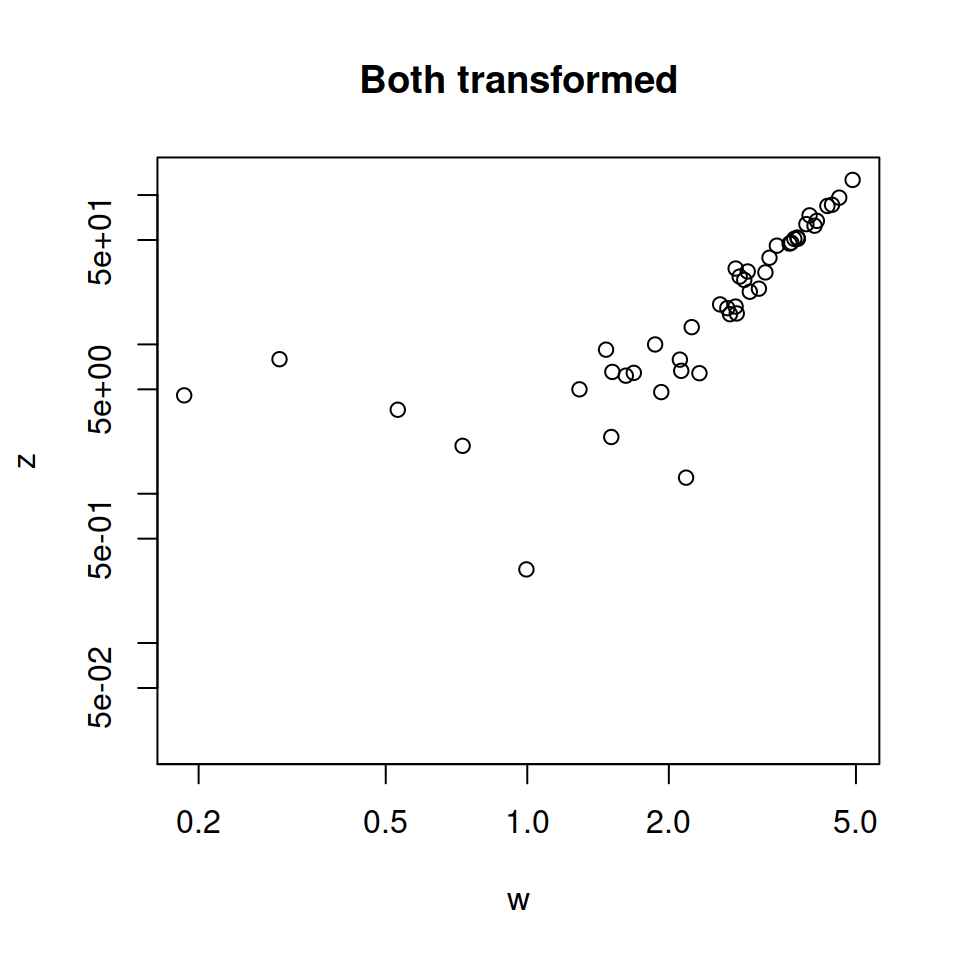Both transformed.

``````# Log scale. X and Y axis
plot(w, z,
log = "xy",
main = "Both transformed")``````

## Dual axis

You can join together two plots with different Y-axis scale increasing the margins of the plot, using `par(new = TRUE)`, creating a new plot without axis or labels and setting a new axis with the `axis` function.

``````# Increase the plot margins
par(mar = c(5, 4, 4, 4) + 0.25)

# Data
plot(x, y, pch = 19, ylab = "Var 1")

# Needed to merge the plots
par(new = TRUE)

# More data
plot(x ^ 2, y ^ 2,
col = 4, pch = 19,
axes = FALSE, # No axes
bty = "n",    # No box
xlab = "", ylab = "") # No axis labels

# New axis
axis(4)
# Axis label
mtext("Var 2", side = 4, line = 3, col = 4)``````